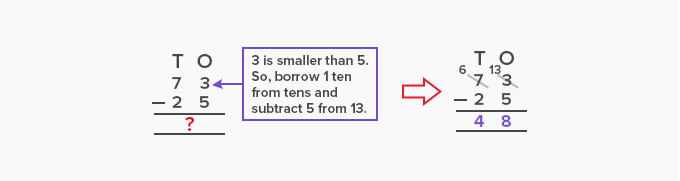# Subtrahend - Definition with Examples

The Complete K-5 Math Learning Program Built for Your Child

• 30 Million Kids

Loved by kids and parent worldwide

• 50,000 Schools

Trusted by teachers across schools

• Comprehensive Curriculum

Aligned to Common Core

What is Subtrahend?

The number which we subtract from another number in a subtraction sentence is called a subtrahend.

A subtraction sentence consists of 3 numbers:

Minuend, Subtrahend and Difference

Subtrahend is the second number in a subtraction sentence. It is subtracted from the minuend to get the difference.In the vertical method of subtraction or column method, the subtrahend is the number above the difference.In the column method of subtraction, the subtrahend is usually smaller than the minuend. However, if the minuend is lower than the subtrahend, we perform regrouping or borrowing. It makes the value of minuend higher than the subtrahend and makes it possible to subtract the subtrahend from the minuend.In the above subtraction, we cannot subtract 5 from 3. So, we borrow 1 ten from the tens place. It changes the minuend from 3 to 13. Now, we can easily subtract 5 from 13 to get 8.

 Fun Facts The word subtrahend originated in the late 17th century from the Latin word subtrahendus, which means to be taken away. In a subtraction sentence, if the value of the subtrahend is missing and the minuend and difference are known, then we can find the subtrahend by subtracting the difference from the minuend. For example, In 56 – ­­­­­­­_____ = 34; the subtrahend is 56 – 34 = 22.

Won Numerous Awards & Honors